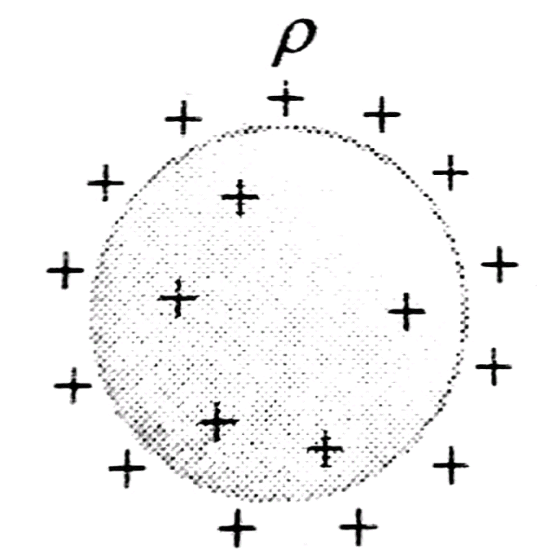Q

# Need clarity, kindly explain! - Charge is distributed within a sphere of radius R with a volume charge density , where A and a are c - Electrostatics - JEE Main

Charge is distributed within a sphere of radius R with a volume charge density , where A and a are constants. If Q is the total charge of this charge distribution, the radius R is:

• Option 1)

• Option 2)

• Option 3)

• Option 4)

Views

Volume Charge distribution -

charge per unit volume.

- wherein(charge on a dielect ric sphere etc)Option 1)

Option 2)

Option 3)

Option 4)

Exams
Articles
Questions